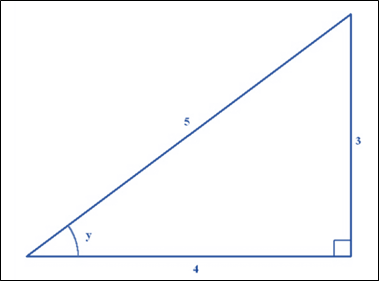# The value of following expression: cos 2 y### Single Variable Calculus: Concepts...

4th Edition
James Stewart
Publisher: Cengage Learning
ISBN: 9781337687805### Single Variable Calculus: Concepts...

4th Edition
James Stewart
Publisher: Cengage Learning
ISBN: 9781337687805

#### Solutions

Chapter C, Problem 28E
To determine

## To calculate: The value of following expression:   cos2y

Expert Solution

The given expression cos2y is evaluated as 725 .

### Explanation of Solution

Given information:

sinx=13secy=540x,yπ2

Formula Used:

cos2θ=2cos2θ1

Calculation:

Know that:

Need to sketch the triangle for secx :Fig. 2 Triangle for secx

So, hypotenuse is equal to 5 and the length of the adjacent side is equal to 4 . So, use the Pythagoras theorem:

r2=opposite2+a252=opposite2+42opposite2=9opposite=3

And the remaining side opposite to y is 3 . :

siny=35cosy=1secy=45

Now, evaluate the given expression as:

cos2y=2cos2y1cos2y=2(45)21cos2y=2(1625)(2525)cos2y=(3225)(2525)cos2y=322525cos2y=725

### Have a homework question?

Subscribe to bartleby learn! Ask subject matter experts 30 homework questions each month. Plus, you’ll have access to millions of step-by-step textbook answers!